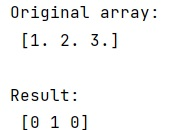# What is "NumPy TypeError: ufunc 'bitwise_and' not supported for the input types" when using a dynamically created boolean mask, how to fix it?

Learn about the "NumPy TypeError: ufunc 'bitwise_and' not supported for the input types" when using a dynamically created boolean mask, and its solution. By Pranit Sharma Last updated : April 05, 2023

Suppose that we are given a numpy array of floats and we are creating a mask from this array where this array equals to a particular value, if yes, we do a bitwise AND (&) operation of this value with some other value.

This can be done in a single-line statement as:

```arr == 'some value' & b
```

But this statement generates a TypeError as "ufunc 'bitwise_and' not supported for the input types", and the inputs could not be safely coerced to any supported types according to the casting rule ''safe'. We get this error because bitwise_and is not defined for a floating-point scalar and a boolean array.

## How to fix "ufunc 'bitwise_and' not supported for the input types" TypeError?

To fix NumPy TypeError: ufunc 'bitwise_and' not supported for the input types, we need to separate the expression by adding a parathesis to the statement. We can also do this by converting the float array into an int array.

Let us understand with the help of an example,

## Python code to "ufunc 'bitwise_and' not supported for the input types" TypeError

```# Import numpy
import numpy as np

# Creating an array
arr = np.array(np.array([1.0, 2.0, 3.0]))

# Display array
print("Original array:\n",arr,"\n")

arr = (arr == 2.0) & 3

# Display result
print("Result:\n",arr,"\n")
```

### Output

The output of the above program is: